## Commonly used Op-Amp ICs and How to Select One for Your Next Application

One of the most important functions of electronic circuitry is amplification. In the absence of amplification, many other specific circuits would not work. For example, oscillators to produce sine waves, square waves, pulse or any other desired wave shapes would not be possible without amplifier circuits.

The operational amplifier or op-amp enables a very high-performance and highly stable amplification circuit with very few passive components, so in this tutorial, we are going to know about the most commonly used Op-amp ICs and how to choose an op-amp for your application. We have already covered a wide range of op-amp ICs with their description, pinouts, and working.

### What is an Op-Amp?

Before we go any further, let us discuss the basics of op-amps. If we imagine a perfect amplifier, it should have infinite gain, infinite input impedance, zero output impedance, and infinite bandwidth but in real-life scenarios, nothing is ideal so they have finite gain and finite bandwidth.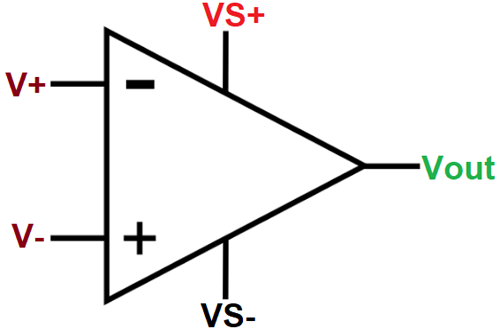The term operational amplifier was originally used for the DC amplifiers that perform mathematical operations as summation, subtraction, integration, and differentiation in analog computers. The scope of operational amplifiers is much wider and can be put to a variety of other than amplification, e.g. voltage regulators in instrumentation and control systems.

An operational amplifier can amplify signals having a frequency ranging from 0 Hz to 1 MHz This means that OP-AMP can be used to amplify DC signals (0 Hz) as well as AC input signals (high-frequency signals). Furthermore, it is designed in such a way that external components like resistors, capacitors, etc. can be connected to its terminals. And by adding these passive components, its external characteristics can be changed.

### Understanding Op-amp Parameters

While designing a product, it is essential to know about the characteristics of an OP-Amp, i.e. if you want to design a product efficiently and keep the cost to a minimum, then you should learn about the following parameter of an op-amp:

1. Open Loop Voltage Gain: The most important function of an amplifier is to amplify a signal, and every amplifier has some gain. It means if an input voltage is applied, it will be multiplied by the gain. For example, if an Op-Amp has a gain of 2*10^5 and the input is 2 V, we will have an output voltage of 400,000V, practically it's not possible because it means that the Op-Amp is SATURATED and the output voltage is limited to VCC.

2. Input Resistance: It is the resistance between the non-inverting and the inverting input of the amplifier.

3. Output Resistance: The output resistance is like the internal resistance of the battery, and hence it is the very small resistance, which means the output voltage is dependent on the load.

4. Input Offset Voltage: If we apply the same input voltage in both terminals of the Op-Amp, the output should be zero volts. Practically, it’s not possible because the transistors inside do not have the same value, so a very small voltage appears at the output. So, to make the output zero, we have to apply a small differential voltage, this voltage is known as the input offset voltage.

5. Input Offset Current: If we connect both the non-inverting and inverting terminal of the Op-Amp to ground, we can see a very small amount of current will flow through the output pin of the Op-Amp, this current is produced because the base current of the internal transistor is not the same, so to make the output current to zero a small input current is applied to cancel out the output current, this input current is known as the Input Offset Current.

6. Input Bias Current: The input bias current is the average of the currents that flow into the inverting and non-inverting input terminals of the Op-Amp. The smaller the input bias current, the smaller the drift.

7. CMRR: The Common Mode Rejection Ratio (CMRR) is defined as the ratio of the differential voltage gain to the common-mode voltage gain. The ability of a differential amplifier to reject a common-mode signal is expressed by the Common Mode Rejection Ratio.

8. Slew Rate: It is defined as the maximum rate of change of output voltage per unit of time and expressed in volts per microsecond. Slew rate indicates how fast the output of OP-AMP can change in response to change in input frequency

9. Voltage Swing: It is defined as how close the output voltage can reach the supply voltage or how close the output can be driven to rail to rail.

10. Gain-Bandwidth Product: We know that due to parasitic junction capacitance and minority-carrier change storage in devices, the voltage gain of OP-amp decreases at high frequencies. As the input signal of the frequency increases, the open-loop gain drops off until it finally reacts to the value 1. The frequency at which the gain reduces to 1 is defined as unity gain frequency or unity-gain bandwidth.

11. Peak-to-Peak Input Noise Voltage: If we look at a noise signal it will have a Max-point and a Min-Point and the difference between the min and max is known as the Peak-to-Peak Input Noise Voltage.

12. Unity Gain: A Unity gain amplifier is an amplifier that has a gain of 1 that also means there is no gain. The output voltage will be the same as the input voltage it is commonly known as a voltage follower amplifier.

Now we will discuss about different types of Op-amp ICs that can be useful for your next amplifier design.

### 1. uA709M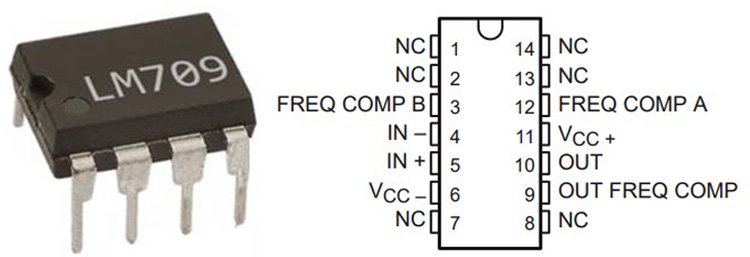The LM709 is an industrial classic, a mono amplifier designed and manufactured by Texas instruments in 1971 for general-purpose applications. It comes in an 8-pin DIP, 10-pin DIP, 14-pin DIP package and offers interesting features which are discussed below

• It has a max supply voltage of ±18 V
• It has a max input voltage of ±10 V
• It has a low offset voltage of 6mv max and can drift 6 µV/°C
• It has an input offset current of 500nA max and can drift upto 22.8 nA/°C
• It has an input resistance of 750K
• It has an output resistance of 150Ω
• Common mode rejection ratio (CMRR) is 70 dB max
• Maximum peak-to-peak output voltage swing is 24V
• Operating temperature range –55 to 125 °C

Applications include voltage follower, basic comparator, multivibrator & frequency generator.

Part Number: uA709M

Similar Products: LM709

### 2.  LM741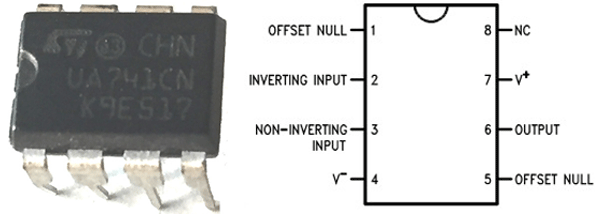The LM741 is another classic monolithic amplifier designed and manufactured by Texas Instruments in 1981 for general-purpose applications. It comes in 8-pin PDIP (Plastic Dual Inline Package), 8-pin CDIP (Ceramic Dual Inline Package) & TO-99 packages and offers interesting features which are discussed below

• It has a max supply voltage of ±22 V
• It has a max input voltage of ±15 V
• It has a voltage gain of 200V/mV
• It has built-in output short circuit protection
• It has a low offset voltage of 6mv max and can drift 15 µV/°C
• It has an input offset current of 70nA max and can drift up-to 0.5 nA/°C
• It has an input resistance of 6M
• Output short circuit current is 40 mA max
• Common mode rejection ratio (CMRR) is 90 dB max
• Maximum peak-to-peak output voltage swing is16V max
• Bandwidth is 1.5Mhz Max
• Slew rate is 0.7 V/us Max
• Operating temperature range –50 to 125 °C

Applications of LM741 include Comparator, DC Amplifier, Summing Amplifier, Integrator or Differentiators and Active Filters.

Part Number: LM741

Similar Products: µA741

### 3.  LM1458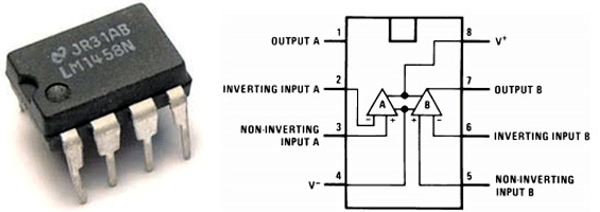The LM1458 is a single supply, high gain, internally frequency compensated dual Op-Amp designed and manufactured by Texas Instruments in 1998 for general-purpose applications. It comes with various packages like TO-CAN, DSBGA(Die-Size Ball Grid Array), SOIC(Small Outline Integrated Circuit) and PDIP(Plastic Dual Inline Package) package. It also offers interesting features, which are discussed below:

• It has a max supply voltage of ±18 V
• It has a max input voltage of ±15 V
• It has a voltage gain of 15V/mV
• It has built-in output short circuit protection
• It has a low offset voltage of 6mv max and can drift 15 µV/°C
• It has an input offset current of 300nA max and can drift up-to 0.5 nA/°C
• It has an input resistance of 1
• Common mode rejection ratio (CMRR) is 90 dB max
• Maximum peak-to-peak output voltage swing is 14V max
• Bandwidth is 1 MHz, Max
• Operating temperature range 0 to 70 °C

Applications of LM1458 include summing amplifiers, portable devices, Comparators, Integrators, etc.

Part Number: LM1458

Similar Products: MC1458

### 4. LM358N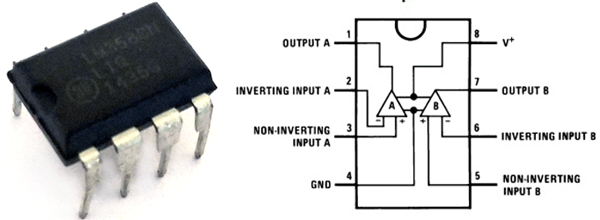The LM358N is a single supply, high gain, internally frequency compensated dual Op-Amp designed and manufactured by Texas Instruments in 2000 for general-purpose application. It comes in various packages like TO-CAN, DSBGA (Die-Size Ball Grid Array), SOIC (Small Outline Integrated Circuit) and PDIP (Plastic Dual Inline Package) packages and offers interesting features which are discussed below:

• It has a max supply voltage of 32 V
• It has a voltage gain of 100 V/mV
• It has continuous output short circuit protection
• It has a low offset voltage of 5mv max and can drift 7µV/°C
• It has an input offset current of 100nA max and can drift up-to 10 pA/°C
• It has an input resistance of 10MΩ
• Output short circuit current is 60 mA max
• It has an output resistance of 300Ω
• Common mode rejection ratio (CMRR) is 70 dB max
• Maximum peak-to-peak output-voltage swing is16V max
• Bandwidth is 1 MHz
• Slew rate is 0.9 V/us Max
• Operating temperature range 0 to 70 °C

Application of LM358N includes Active Filters, General Signal Conditioning and Amplification, 4- to 20-mA Current Loop Transmitter, Uninterruptible power supplies, Programmable logic controllers, etc.

Part Number: LM358N

Similar Products: LM358B, LM358(ST)

### 5. LM324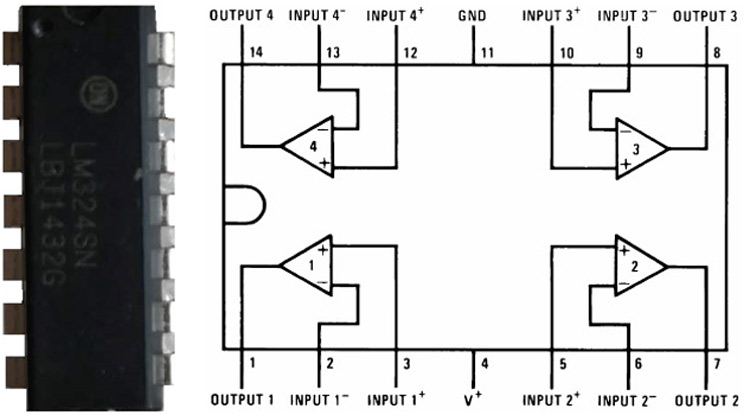The LM324N is a single supply, high gain, internally frequency compensated quad Op-Amp designed and manufactured by Texas Instruments in 2000 for general-purpose applications, It comes in 14-pin PDIP (Plastic Dual Inline Package), 14-pin CDIP(Ceramic Dual Inline Package), 14-pin SOIC(Small Outline Integrated Circuit) & 14-pin TSSOP(Thin-shrink small outline package) packages and offers interesting features which are discussed below

• It has a max supply voltage of 32 V
• It has a voltage gain of 100 V/mV
• It has an input bias current of 100nA
• It has built-in output short circuit protection
• It has a low offset voltage of 3mv max and can drift 30µV/°C
• It has an input offset current of 30nA max and can drift up-to 300 pA/°C
• Output short circuit current is 60 mA max
• It has an output resistance of 350Ω
• Common mode rejection ratio (CMRR) is 85 dB max
• Maximum peak-to-peak output voltage swing is16V max
• Bandwidth is 1 MHz
• Operating temperature range 0 to 70 °C

Part Number: LM324

Similar Products: LM324(ON Semiconductors)

### 6. LF353-N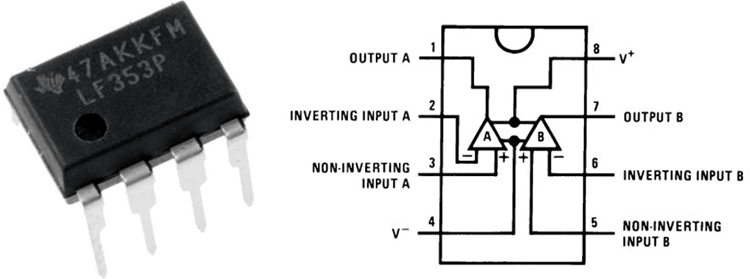The LF353-N is a low-cost dual supply, high gain, high bandwidth, dual JFET input operational amplifiers with an internally trimmed input offset voltage, a dual Op-Amp designed and manufactured by Texas Instruments in 1998 for general purpose system-critical applications. It comes in 8-pin PDIP (Plastic Dual Inline Package) package and offers very interesting features which are discussed below

• It has a max supply voltage of ±18 V
• It has a voltage gain of 100 V/mV
• It has an input bias current of 200pA
• It has built-in output short circuit protection
• It has a low offset voltage of 10mv max and can drift 10µV/°C
• It has an input offset current of 100pA max and can drift up-to 5 pA/°C
• It has an input resistance of 10^12Ω
• It has an output resistance of 350Ω
• It has a voltage swing of ±13.5 V
• Common mode rejection ratio (CMRR) is 100 dB max
• Maximum peak-to-peak output voltage swing is16V max
• Gain Bandwidth Produce is 4 MHz
• Slew rate is 13 V/us Max
• Operating temperature range 0 to 150 °C

Applications of LF353-N include high-speed integrators, Fast D/A converters, sample and hold circuit

Part Number: LF353-N

Similar Products: LF353(ON Semi), LF353(ST)

### 7. TL074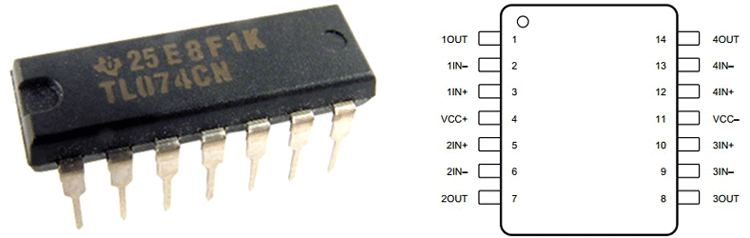The Tl074 is a low-cost dual supply, high gain, high bandwidth, dual JFET input operational amplifiers with an internally trimmed input offset voltage. It is a quad Op-Amp designed and manufactured by Texas Instruments in 1978 for high-speed applications. It comes in 14-pin PDIP (Plastic Dual Inline Package) 14-pin CDIP (Ceramic Dual Inline Package), 14-pin SOIC (Small Outline Integrated Circuit) & 14-pin TSSOP (Thin-shrink small outline package) packages and offers interesting features which are discussed below

• It has a max supply voltage of ±15 V
• It has a supply current of 2.5 mA maximum for each Op-Amp
• It has an input bias current of 200pA
• It has built-in output short circuit protection
• It has a low offset voltage of 13 mv max and can drift 18µV/°C
• It has an input offset current of 200pA
• Output short circuit current is 30 mA max
• It has an input resistance of 10^12 Ω
• Common mode rejection ratio (CMRR) is 100 dB max
• Maximum peak-to-peak output voltage swing is13.5 V max
• Bandwidth is 3 MHz
• Slew rate is 13 V/us
• It has a total harmonic distortion: 0.003%(Typical)
• Operating temperature range 0 to 70 °C

Applications of Tl074 include oscillators, High-Q Notch Filter, Square-Wave Oscillator. It’s very low current consumption leads to battery-powered applications, Unity Gain Amplifier, Audio Tone control circuit and many more.

Part Number: TL074xN

Similar Products: TL082, TL084

### 8. NE5532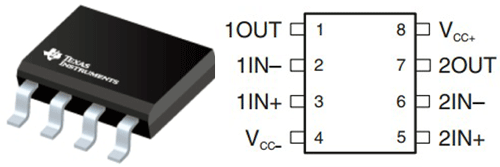The NE5532 is a low-cost, dual supply a high-performance amplifier with exceptionally good performance, designed and manufactured by Texas Instruments in 1979 for efficient budget applications. It comes in 8-pin PDIP(Plastic Dual Inline Package) 8-pin SOIC(Small Outline Integrated Circuit) packages and offers interesting features which are discussed below

• It has a max supply voltage of ± 15 V
• It has a supply current of 10 mA maximum for each Op-Amp
• It has an input bias current of 1000nA
• It has built-in output short circuit protection
• It has a low offset voltage of 5 mV
• It has an input offset current of 200nA
• Output short circuit current is 60 mA max
• It has an input resistance of  300KΩ
• It has an output resistance of 0.3Ω
• Common mode rejection ratio (CMRR) is 100 dB max
• Maximum peak-to-peak output voltage swing is 26 V max
• Bandwidth is 10 Mhz Max
• Slew rate is 9 V/us Max
• Operating temperature range -65 to 150 °C

Applications of NE5532 include Av Receivers, Audio mixer, High-performance audio preamplifier and many more.

Part Number: NE5532A

Similar Products: NE5532(ON Semi), 5962-9760301QPX

### 8. OP07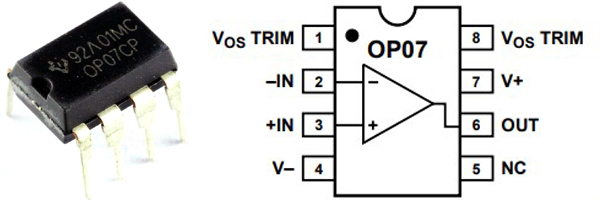The OP07 is a dual supply, high gain, precision operational amplifier with ultra-low offset voltage designed and manufactured by Analog Devices in 2002 for high precision applications. It comes in 8-pin PDIP (Plastic Dual Inline Package), 8-pin SOIC (Small Outline Integrated Circuit) & TO-99 packages and offers very interesting features which are discussed below:

• It has a max supply voltage of ± 15 V
• It has a voltage gain of 450 V/mV
• It has a supply current of 4 mA maximum for each Op-Amp
• It has an input bias current of 7nA
• It has built-in output short circuit protection
• It has a low offset voltage of 75 μV max and can drift 1.3 µV/°C
• It has an input offset current of 3.8nA and can drift up to 35pA/°C
• It has an input resistance of 50MΩ in common mode and 160 GΩ in differential mod
• It has an output resistance of 60 Ω
• Common mode rejection ratio (CMRR) is 106 dB max
• Maximum peak-to-peak output voltage swing is 12.6 V max
• Bandwidth is 0.6 MHz Max
• Slew rate is 0.3 V/us Max
• Operating temperature range -40 to 85 °C

Its applications include Absolute Value Circuit, Low-Frequency Noise Circuit, High Speed, Low VOS Composite Amplifier, Adjustment-Free Precision Summing Amplifier, High Stability Thermocouple Amplifier, Precision Absolute-Value Circuit and many more.

Part Number: OP07EPZ

Similar Products: OP07CP (Texas Instruments)

### 9. MAX4238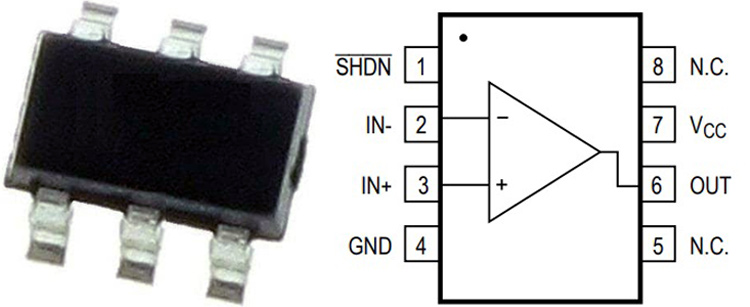The MAX4238 is costly low noise, high gain, high bandwidth, ultra high precision CMOS amplifier designed and manufactured by maxim integrated for very high precision applications. It comes in SO6 and SOT23 (small-outline transistor) packages and offers interesting features which are discussed below

• It has a max supply voltage of 6 V
• It has a supply current of 600µA
• It has an input bias current of 1 pA
• It has a continuous output short circuit duration
• It has a low offset voltage of 2 µV
• It has an input offset current of 2 pA
• Output short circuit current is 40 mA max
• Common mode rejection ratio (CMRR) is 140 dB max
• Maximum peak-to-peak output voltage swing is 50 mV@RL=10K
• Bandwidth is 1 MHz, Max
• Slew rate is 0.35 V/us Max
• Operating temperature range -40 to 150 °C

Applications of MAX4238 include Thermocouple, Strain Gauges, Electronic Scales, Medical Instruments, Instrumentational Amplifier, etc.

Part Number: MAX4238AUT-T

Similar Products: MAX4239

### Conclusion

For selecting the right op-amp for your application, you should go through datasheets of different op-amps and pick one whose specifications meet your project requirement. Choosing an Op-Amp just by assumption can ruin your day.

## Related Post

### Join 20K+subscribers

We will never spam you.

* indicates required

Be a part of our ever growing community.